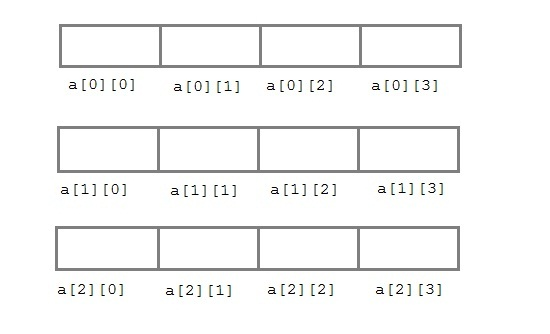# Types of arrays in C

## One dimensional array

One dimensional array – A normal array with ‘n’ elements can also called as 1-D array where it has only one row and ‘n’ elements.

## Multidimensional array• Multi-dimensional arrays are declared by providing more than one set of square [ ] brackets after the variable name in the declaration statement.
Example:-
`int darr // One for column and one for row`
• One dimensional arrays do not require the dimension to be given if the array is to be completely initialized.  By analogy, multi-dimensional arrays do not require the first dimension to be given if the array is to be completely initialized.  All dimensions after the first must be given in any case.

• For two dimensional arrays, the first dimension is commonly considered to be the number of rows, and the second dimension the number of  columns.  We will use this convention when discussing two dimensional arrays.

• Two dimensional arrays are considered by C/C++ to be an array of ( single dimensional arrays ).  For example, "int numbers[ 5 ][ 6 ]"  would refer to a single dimensional array of 5 elements, wherein each element is a single dimensional array of 6 integers.  By extension, "int numbers[ 12 ][ 5 ][ 6 ]" would refer to an array of twelve elements, each of which is a two dimensional array, and so on.

• Another way of looking at this is that C stores two dimensional arrays by rows, with all elements of a row being stored together as a single unit.  Knowing this can sometimes lead to more efficient programs.

• Multidimensional arrays may be completely initialized by listing all data elements within a single pair of curly {} braces, as with single dimensional arrays.

• It is better programming practice to enclose each row within a separate subset of curly {} braces, to make the program more readable.This is required if any row other than the last is to be partially initialized.  When subsets of braces are used, the last item within braces is not followed by a comma, but the subsets are themselves separated by commas.

• Multidimensional arrays may be partially initialized by not providing complete initialization data.  Individual rows of a multidimensional array may be partially initialized, provided that subset braces are used.

• Individual data items in a multidimensional array are accessed by fully qualifying an array element.

• Alternatively, a smaller dimensional array may be accessed by partially qualifying the array name.

• For example, if  "data" has been declared as a three dimensional array of floats, then data[ 1 ][ 2 ][ 5 ] would refer to a float, data[ 1 ][ 2 ] would refer to a one-dimensional array of floats, and data[ 1 ] would refer to a two-dimensional array of floats.

• The reasons for this and the incentive to do this relate to memory-management issues that are beyond the scope of these notes.

## Example of 2D array

This program demonstrates how to store the elements entered by user in a 2d array and how to display the elements of a two dimensional array.